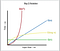Photo by Joshua Aragon on Unsplash

This is an important topic in the coding world. I was introduced to this concept early on in my journey with code, but it went right over my head. I saw graphs and numbers and math I’d never seen before. Fortunately, as I continued to learn, I’ve come to realize that the math isn’t as complicated as it may seem. In a nutshell, and I will expand on this: Big O, is just a way of generalizing the complexity of functions. The purpose of this blog is to help others make the first “big” leap into understanding what Big O is.

We’ll start with WHAT Big O is, then move onto HOW Big O works, and end with WHY we use Big O.

What is Big O?

Again, Big O is a way to determine the efficiency of our code. It is a system that allows us to compare and generalize code, which then makes it possible for us to talk about and analyze the performance of code. Let us assume that as the number of our input increases, so will the runtime of our code. However, there can be many implementations or many ways of writing out the same code.

So let’s get the Big Elephant out of the room first. Knowing that we can have multiple implementations of the same code… Big O wants to know, “What is the best implementation?” or “What is the most efficient implementation?” What implementation would consistently run the quickest if our input would increase? We don’t care about how long a computer takes to run the code, we care about the general trend and behavior of the code. Generally, what performs better as our input increases? What we’re observing is, how the runtime of an algorithm grows as the input grows. Does it grow exponentially, gradually or linearly, or is it constant?

How does Big O work?Big O Time Complexity Graph. Credit: Huang, D. (2018, January 1). Javascript — Algorithm

It gives us a numeric representation of code performance. Instead of counting seconds, we count the number of simple operations in the code. In other words, when you consider the input, what we are counting in terms of performance, is how the input behaves inside the function. Take for instance a function that finds the sum of two numbers in an array that is equal to a target that is inserted as the second argument of the same function.

Let’s keep our focus to three types of O performance: Linear, Quadratic, and Constant.

A Linear algorithm will increase in time as the input increases. This can be seen in a function with a standard loop for instance. Where the input equals an array with a length of 4 indexes, the worst case runtime of that function is 4 total iterations through this loop.
A Quadratic algorithm will increase to the power of 2 as the input increases. This response can be observed in functions with nested loops. Because as an input enters the first loop, it then goes through a second loop and must iterate completely through that second loop before moving back out into the first loop and repeating this process. So if we had an array with a length of 4 indexes enter a function with one nested loop, in the worst case, it would have to go through a total of 16 iterations before completing.
And lastly a Constant algorithm will return a constant value regardless of our input. So for instance if we are just setting our input equal to 5, the equal operator would set our performance to O(1). This algorithm will always return the same value, even if our input were to increase.

Why do we use Big O?

There are many ways to write the same code, and knowing the performance of our code gives us a preciseness in how we can talk about the performance of code. It gives us the language to speak about and identify inefficiencies in code. Thus in conclusion, Big O is an abstraction that simplifies how we can discuss code in order to improve run time of code. It helps bring an abstract idea down to a general understanding so that we can find the best possible implementations of our code. Understanding Big O is important and one of the many starting points to better understanding code.

A Software Engineer with a background in Education Technology and Dance. Recent grad form FlatIron Bootcamp, and passion for the arts and working with databases

## More from Stephen Galvan

A Software Engineer with a background in Education Technology and Dance. Recent grad form FlatIron Bootcamp, and passion for the arts and working with databases